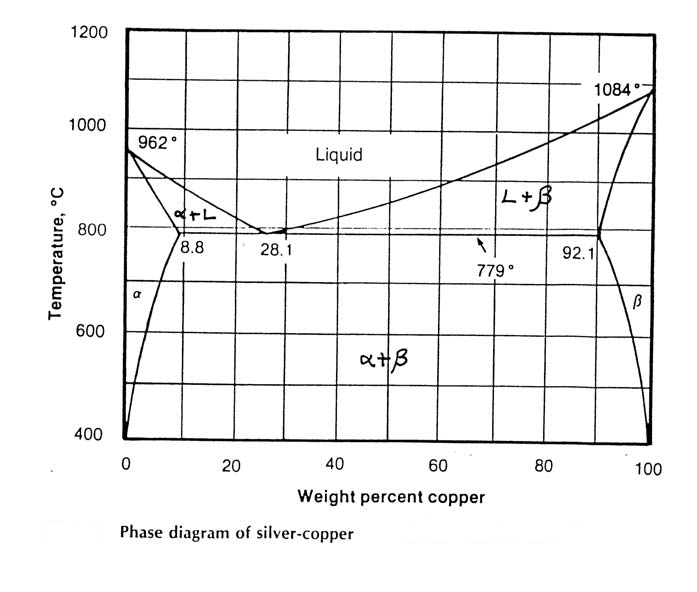### Materials chemistry find potential energy between two atoms

Assignment Help Chemical Engineering
##### Reference no: EM131322

1. The potential energy between two atoms can be represented as follows:

V = -A/r + B/r10

Where A and B are constants and r the interatomic separation distance. Plot the potential energy verses distance relationships for these atoms when A= 8 x 10-30 J.m and B= 5 x 10-119 J.m10. Determine the coefficient of thermal expansion (in m/m.K) for the material over the following temperature ranges:
a) 0oK to 0oC,
b) 0oC to 820oC.
assume that thermal energy of the system is 3kT/2 where k is Boltzman's constant k = 1.381 x10-23 J/K.

2. Gallium arsenide (GaAs) has the zinc blende structure. The gallium atoms are in FCC packing and the arsenic atoms occupy half the tetrahedral sites. Calculate the density of Gallium arsenide (GaAs) using the following data.

Ga        1.26 Ångstroms               69.7 g/mole
As        1.19 Ångstroms                74.9 g/mole

Avogadro's number (NAv) is 6.02 x 1023 atoms per mole.

3. Using the phase diagram below for the copper-silver system, determine:

i) the phases present

ii) the composition (wt % Cu) of those phases and

iii) the amount (wt %) of each phase for

a) a copper-silver alloy (70 wt % copper) at 900oC

b) a copper-silver alloy (70 wt % copper) at 600oC

c) a copper-silver alloy (5 wt % copper) at 800oC### Write a Review

#### Why a substance heated at a fast rate

Why a substance is being heated at a fast rate the temperature of decomposition

#### Draw t-s diagram of the cycle

Draw T-S diagram of the cycle.

Application of reverse osmosis principles for the desalination of sea water

#### Baffled tank with a six blade turbine agitator

Equal rates of mass transfer for the production of the fine chemical are required. This is often required for certain types of organic synthesis.

#### Evaluation of a new chemical manufacturing process

Prepare the design and evaluation of a new chemical manufacturing process.

#### Design variables necessary for sizing equipment

Assist with the setting of design variables necessary for sizing equipment

#### Solve the initial value problem

Use Laplace transformation to solve the initial value problem